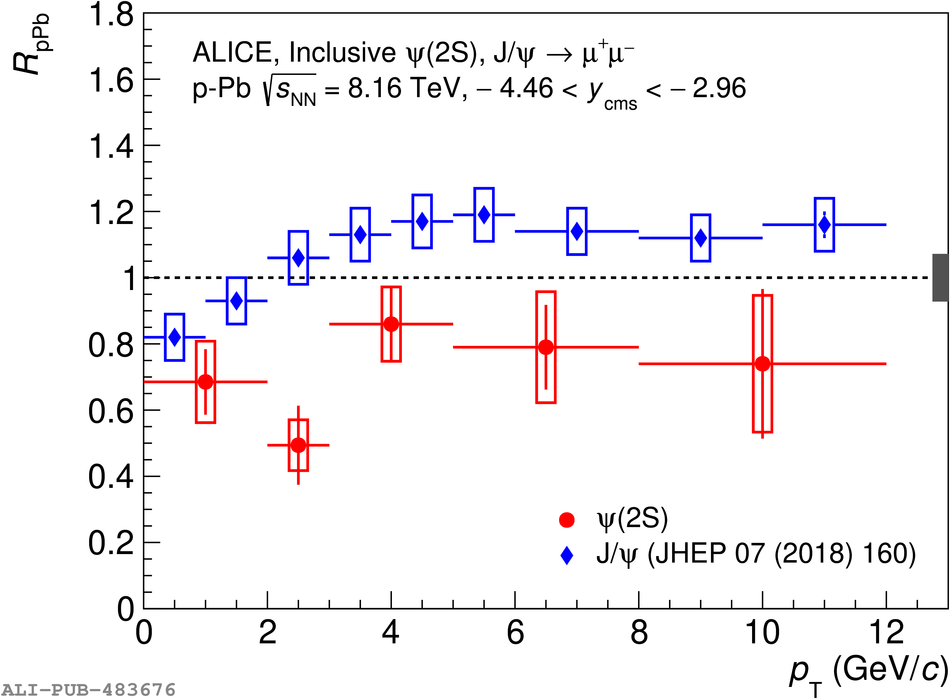# Figure 7

 The $p_{\rm T}$-dependence of $R_{\rm{pPb}}$ for \psip and \jpsi at forward (left) and backward (right) rapidity in \ppb collisions, at $\sqrtSnn = 8.16$ TeV. The error bars represent the statistical uncertainties, while the boxes correspond to uncorrelated systematic uncertainties and the box at $R_{\rm pPb}=1$ to correlated systematic uncertainties. The comparison with the results of a CGC-based model , which implements final-state effects, is also shown.